## 3.1 Introduction

Classification is the process where a model or classifier is constructed to predict categorical labels of unknown data. Classification problems aim to identify the characteristics that indicate the group to which each case belongs. This pattern can be used both to understand the existing data and to predict how new instances will behave.

Definition: Classification is the task of learning a target function f that maps each attribute set X to one of the predefined class label Y.

For example, classification of loan applicants as “safe” or “risky” for the bank, whether a customer with a given profile will buy a new computer or not, whether a patient is a good candidate for a surgical procedure or not etc.

Data classification is a two-step process:

I. Model construction
In the first step, a classifier is built describing a predetermined set of data classes or concepts. This is the learning step (or training phase), where a classification algorithm builds the classifier by analyzing or “learning from” a training set made up of database tuples and their associated class labels.

II. Model usage
In the second step, the model is used for classification. Test data are used to estimate the accuracy of the classification rules. If the accuracy is considered acceptable, the rules can be applied to the classification of new data tuples.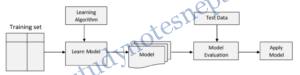Example: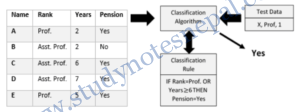### Issues Regarding Classification

i. Preparing the Data for Classification

Data cleaning: This refers to the preprocessing of data in order to remove or reduce noise (by applying smoothing techniques, for example) and the treatment of missing values (e.g., by replacing a missing value with the most commonly occurring value for that attribute, or with the most probable value based on statistics).

Relevance analysis: Many of the attributes in the data may be redundant. Correlation analysis can be used to identify whether any two given attributes are statistically related. For example, a strong correlation between attributes A1 and A2 would suggest that one of the two could be removed from further analysis.

Data transformation and reduction: The data may be transformed by normalization, particularly when neural networks or methods involving distance measurements are used in the learning step. Normalization involves scaling all values for a given attribute so that they fall within a small specified range, such as -1.0 to 1.0, or 0.0 to 1.0. Data can also be reduced by applying methods such as binning, histogram analysis, and clustering.

ii. Evaluating Classification Methods
Classification methods can be compared and evaluated according to the following criteria:

Accuracy: The accuracy of a classifier refers to the ability of a given classifier to correctly predict
the class label of new or previously unseen data (i.e., tuples without class label information).

Speed: This refers to the computational costs involved in generating and using the given classifier or predictor.

Robustness: This is the ability of the classifier or predictor to make correct predictions given noisy data or data with missing values.

Scalability: This refers to the ability to construct the classifier or predictor efficiently given large amounts of data.

Types of Classifiers:

• Decision Tree classifier
• Rule Based Classifier
• Nearest Neighbor Classifier
• Bayesian Classifier
• Artificial Neural Network (ANN) Classifier

## 3.2 Decision Tree Classifier

Decision tree is a collection of decision nodes, connected by branches, extending downward from the root node until terminating in leaf nodes. Beginning at the root node, which by convention is placed at the top of the decision tree diagram, attributes are tested at the decision nodes, with each possible outcome resulting in a branch. Each branch then leads either to another decision node or to a terminating leaf node.

The problem of constructing a decision tree can be expressed recursively. First, select an attribute to place at the root node and make one branch for each possible value. This splits up the example set into subsets, one for every value of the attribute. Now the process can be repeated recursively for each branch, using only those instances that actually reach the branch. If at any time all instances at a node have the same classification, stop developing that part of the tree.

“How are decision trees used for classification?” Given a tuple, X, for which the associated class label is unknown, the attribute values of the tuple are tested against the decision tree. A path is traced from the root to a leaf node, which holds the class prediction for that tuple (X). Decision trees can easily be converted to classification rules as well.

The only (and the most important) thing left to decide is how to determine which attribute to split on, given a set of examples with different classes. Consider the weather data in the table 1. There are four possibilities for root node (Outlook, Temperature, Humidity and Wind). Which is the best choice? The number of yes and no classes are shown at the leaves. Any leaf with only one class— yes or no—will not have to be split further, and the recursive process down that branch will terminate. Because we seek small trees, we would like this to happen as soon as possible. If we had a measure of the purity of each node, we could choose the attribute that produces the purest child nodes.

Attribute Selection Measures

An attribute selection measure is an approach for selecting the splitting criterion that “best” separates a given data partition, D, of class-labeled training tuples into individual classes. If we were to split D into smaller partitions according to the outcomes of the splitting criterion, ideally each partition would be pure (i.e. all of the tuples that fall into a given partition would belong to the same class). Conceptually, the “best” splitting criterion is the one that most closely results in such a scenario. Attribute selection measure is also known as splitting rules because they determine how the tuples at a given nodes are to be split.

There are three popular attribute selection measures—information gain, gain ratio, and gini index.

a. Information Gain

Information gain is used by ID3 algorithm. The attribute with the highest information gain is chosen as the splitting attribute for node N. This attribute minimizes the information needed to classify the tuples in the resulting partitions and reflects the least randomness or “impurity” in these partitions.

The expected information needed to classify a tuple in D is given by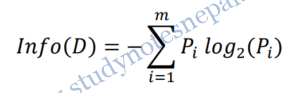Where Pi= probability that an arbitrary tuple D belongs to class Ci

At this point, the information we have is based solely on the proportions of tuples of each class. Info(D) is also known as the entropy of D.

Now, suppose we were to partition the tuples in D on some attribute A having v distinct values, {a1, a2, . . . , aV} as observed from the training data. Attribute A can be used to split D into v partitions or subsets, {D1, D2, . . . , Dv}, where Dj contains those tuples in D that have outcome aj of A. How much more information would we still need (after the partitioning) in order to arrive at an exact classification? This amount is measured by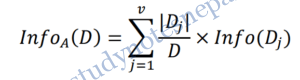|Dj|/D acts as the weight of the jth partition. InfoA(D) is the expected information required to classify a tuple from D based on the partitioning by A.

Finally the information gain of any attribute A can be calculated as: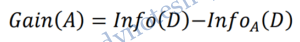In other words, Gain(A) tells us how much would be gained by branching on A. The attribute A with the highest information gain, (Gain(A)), is chosen as the splitting attribute at node N. This is equivalent to saying that we want to partition on the attribute A that would do the “best classification,” so that the amount of information still required to finish classifying the tuples is minimal (i.e., minimum InfoA(D)).

Example 1: Decision tree using information gain.
Table below presents a training set, D, of class-labeled tuples randomly selected from the weather dataset consisting of weather information of last 14 days and whether a match was played on that day or not. Now using the decision tree we need to predict whether the game will happen or not in the day with testing attributes.

In this example, the class label attribute, play_tennis, has two distinct values (yes, no); therefore, there are two distinct classes (i.e., m = 2). Let class C1 correspond to yes and class C2 correspond to no. There are nine tuples of class yes and five tuples of class no. A (root) node N is created for the tuples in D. To find the splitting criterion for these tuples, we must compute the information gain of each attribute.

We first compute the expected information needed to classify a tuple in D as,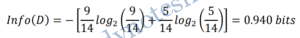Next, we need to compute the expected information requirement for each attribute. Let’s start with the attribute Outlook. We need to look at the distribution of yes and no tuples for each category of age. For the Outlook category sunny, there are two yes tuples and three no tuples (total five). For the category Overcast, there are four yes tuples and zero no tuples (total four). For the category

Rain, there are three yes tuples and two no tuples (total five).
So, the expected information needed to classify a tuple in D if the tuples are partitioned according to Outlook is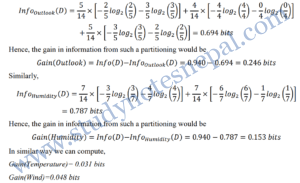Because Outlook has the highest information gain (0.246) among the attributes, it is selected as\ the splitting attribute. Node N is labeled with Outlook, and branches are grown for each of the attribute’s values. The tuples are then partitioned accordingly. So, initially our decision tree will look like: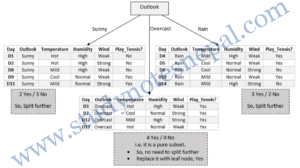Notice that the tuples falling into the partition for Outlook = overcast all belong to the same class. Because they all belong to class “yes,” a leaf should therefore be created at the end of this branch and labeled with “yes”.

As we can see, for Outlook being sunny, there are 2 yes and 3 no. So we have to further split the decision tree. To do so, we need to compute information gain of attributes for the sub table on the left using same methods as above.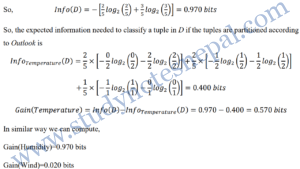So, we select Humidity as splitting criteria. In similar way, we can compute information gain for all attributes in right sub table. In the right sub table, Wind will be our splitting criteria. So our final decision tree will be as follows: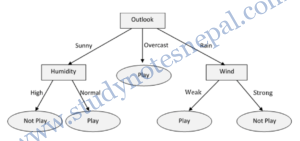b. Gain Ratio
The information gain measure is biased toward tests with many outcomes. That is, it prefers to select attributes having a large number of values. C4.5, a successor of ID3, uses an extension to information gain known as gain ratio, which attempts to overcome this bias. It applies a kind of normalization to information gain using a “split information” value defined analogously with Info(D) as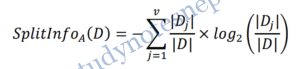This value represents the potential information generated by splitting the training data set, D, into v partitions, corresponding to the v outcomes of a test on attribute A. Note that, for each outcome, it considers the number of tuples having that outcome with respect to the total number of tuples in D. It differs from information gain, which measures the information with respect to classification that is acquired based on the same partitioning. The gain ratio is defined as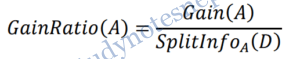The attribute with the maximum gain ratio is selected as the splitting attribute.
For example

A test on Temperature splits the data of Table 1 into three partitions, namely cool, mild, and hot, containing four, six, and four tuples, respectively. To compute the gain ratio of Temperature, we first compute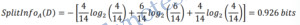From above example, we have Gain(Temperature)=0.031 bits
Therefore, GainRatio(Temperature)=0.031/0.926=0.033 bits.

c. Gini Index
The Gini index is used in CART. Using the notation described above, the Gini index measures the impurity of D, a data partition or set of training tuples, as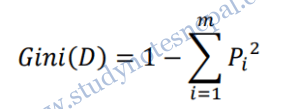Where, pi is the probability that a tuple in D belongs to class Ci and is estimated by |Ci,D|/|D|. The sum is computed over m classes.

The Gini index considers a binary split for each attribute. Let’s first consider the case where A is a discrete-valued attribute having v distinct values, {a1, a2, . . . , av}, occurring in D. To determine the best binary split on A, we examine all of the possible subsets that can be formed using known values of A.

When considering a binary split, we compute a weighted sum of the impurity of each resulting partition. For example, if a binary split on A partitions D into D1 and D2, the gini index of D given that partitioning is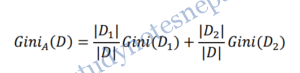For each attribute, each of the possible binary splits is considered. The reduction in impurity that would be incurred by a binary split on a discrete- or continuous-valued attribute A is Gini(A) = Gini(D)-GiniA(D).

—————————– Refer Text Book —————————–

### Tree Pruning

When a decision tree is built, many of the branches will reflect anomalies in the training data due to noise or outliers. Tree pruning methods address this problem of overfitting the data. Such methods typically use statistical measures to remove the least reliable branches. The pruned trees are smaller and less complex. The dual goal of pruning is reduced complexity of the final classifier as well as better predictive accuracy by the reduction of overfitting and removal of sections of a classifier that may be based on noisy data. A tree that is too large risks overfitting the training data and poorly generalizing to new samples.

A small tree might not capture important structural information about the sample space. But it is hard to tell when a tree algorithm should stop because it is impossible to tell if the addition of a single extra node will dramatically decrease error. A common strategy is to grow the tree until each node contains a small number of instances then use pruning to remove nodes that do not provide additional information.

Pruning should reduce the size of a learning tree without reducing predictive accuracy as measured by a test set or using cross-validation.

Approaches:

ii. Pre pruning
In the pre pruning approach, a tree is “pruned” by halting its construction early (e.g. by deciding not to further split or partition the subset of training tuples at a given node). Upon halting, the node becomes a leaf. The leaf may hold the most frequent class among the subset tuples or the probability distribution of those tuples. When constructing a tree, measures such as statistical significance, information gain, Gini index, and so on can be used to assess the goodness of a split. If partitioning the tuples at a node would result in a split that falls below a pre specified threshold, then further partitioning of the given subset is halted. There are difficulties, however, in choosing an appropriate threshold. High thresholds could result in oversimplified trees, whereas low thresholds could result in very little simplification.

ii.  Post pruning
Post pruning removes subtrees from a “fully grown” tree. A subtree at a given node is pruned by removing its branches and replacing it with a leaf. The leaf is labeled with the most frequent class among the subtree being replaced. Post pruning is done by computing the cost complexity. The cost complexity for each internal node N and the complexity if it were to be replaced by a leaf node is computed. If pruning would result in lower cost complexity, it would be pruned.

## 3.3 Rule Based Classifier

Large decision trees are difficult to understand because each node has a specific context established by the outcomes of tests at antecedent nodes. To make a decision tree model more readable, a path to each leaf can be transformed into an IF – THEN production rule. The IF part consists of all tests on a path, and the THEN part is a final classification. Rules in this form are called decision rules. Rules are a good way of representing information or bits of knowledge. An IF-THEN rule is an expression of the form

IF condition THEN conclusion

An example is rule R1,

R1: IF age = youth AND student = yes THEN buys computer = yes.
The “IF”-part (or left-hand side) of a rule is known as the rule antecedent or precondition. The “THEN”-part (or right-hand side) is the rule consequent. In the rule antecedent, the condition consists of one or more attribute tests (such as age = youth, and student = yes) that are logically ANDed. The rule’s consequent contains a class prediction (in this case, we are predicting whether a customer will buy a computer). R1 can also be written as

R1: (age = youth) ˄ (student = yes)) ⇒ (buys computer = yes).

If the condition (that is, all of the attribute tests) in a rule antecedent holds true for a given tuple, we say that the rule antecedent is satisfied (or simply, that the rule is satisfied) and that the rule covers the tuple.

A rule R can be assessed by its coverage and accuracy

• Given a tuple X from a data D
•  ncovers: Number of tuples covered by R
•  ncorrect: Number of tuples correctly classify by R
•  |D|: Number of tuples in D

We can define the coverage and accuracy of R as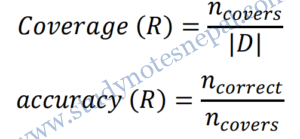For example,
In the training set for buys_computer in table 2, for the rule R1 above,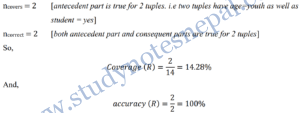### Rule extraction from decision tree

Decision tree classifiers are a popular method of classification. It is easy to understand how  decision trees work and they are known for their accuracy. But decision trees can become large and difficult to interpret.

To extract rules from a decision tree, one rule is created for each path from the root to a leaf node. Each splitting criterion is logically ANDed to form the rule antecedent (IF part). Leaf node holds the class prediction for rule consequent (THEN part).
For example,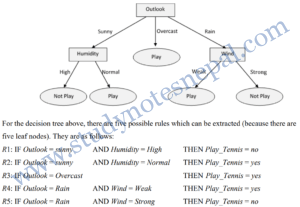## 3.4 Nearest Neighbor Classifier

The nearest neighbor classifier uses the training tuples are stored in an n-dimensional pattern space to classify the testing tuple. A k-nearest-neighbor classifier searches the pattern space for the k training tuples that are closest to the unknown tuple. These k training tuples are the k “nearest neighbors” of the unknown tuple. For k-nearest-neighbor classification, the unknown tuple is assigned the most common class among its k nearest neighbors. When k = 1, the unknown tuple is assigned the class of the training tuple that is closest to it in pattern space. k-nearest neighbor is an example of instance-based learning, in which the training data set is stored, so that a classification for a new unclassified record may be found simply by comparing it to the most similar records in the training set.

Nearest neighbor classifier requires:

• Set of stored records
• Distance matric to compute distance between records. For distance calculation any standard
• approach can be used such as Euclidean distance.
• The value of ‘K’, the number of nearest neighbor to retrieve.

To classify the unknown records:

• Compute distance to other training records.
• Identify the k-nearest neighbor.
• Use class label nearest neighbors to determine the class label of unknown record. In case of conflict, use the majority vote for classification.

For Example,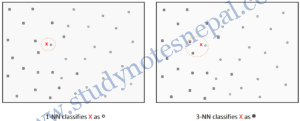Issues with kNN classifier

• Choosing the value of K
• One of challenge in classification is to choose the appropriate value of K. If K is too small, it is sensitive to noise points. If K is too large, neighbor may include points from other classes.
• With the change of value of K, the classification result may vary as in example above.
• Attribute may have to be scaled to prevent distance measure from being dominated by one of attributes. E.g. Height, Temperature etc
• Distance computing for non-numeric data.
• Missing values

• Poor accuracy when data have noise and irrelevant attributes
• Computationally expensive

## 3.5 Bayesian Classifier

Bayesian classifiers are statistical classifiers. They can predict class membership probabilities, such as the probability that a given tuple belongs to a particular class. Bayesian classification is based on Bayes’ theorem, named after Thomas Bayes (1702-1761). Naïve Bayesian classifiers assume that the effect of an attribute value on a given class is independent of the values of the other attributes. Bayesian classifier have minimum error rate compared to all other classifiers. It also has high accuracy and speed for large database.

Bayes Theorem
Let X be a data sample whose class label is unknown. Let H be some hypothesis: such that the data sample X belongs to a specific class C. We want to determine the probability that the hypothesis H holds given the observed data sample X (i.e. P(H|X)). P(H|X) is the posterior probability representing our confidence in the hypothesis after X is given. In contrast, P(H) is the prior probability of H for any sample, regardless of how the data in the sample look. The posterior probability P(H|X) is based on more information than the prior probability P(H). The Bayesian theorem provides a way of calculating the posterior probability P(H|X) using probabilities P(H), P(X), and P(X|H). The basic relation is: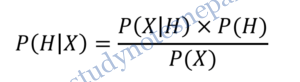Or, the probability that an event H occurs given that another event X has already occurred is equal to the probability that the event X occurs given H has already occurred multiplied by probability that event H occurs divided by probability of occurrence of X.

For example, suppose our world of data tuples is confined to customers described by the attributes age and income, and that X is a 35-year-old customer with an income of \$40,000. Suppose that H is the hypothesis that our customer will buy a computer. Then,

P(H|X) → the probability that customer X will buy a computer given that we know the customer’s age and income. It is the posterior probability, or a posteriori probability, of H conditioned on X.

P(X|H) → the probability that a customer, X, is 35 years old and earns \$40,000, given that we know the customer will buy a computer. It is the posterior probability of X conditioned on H.

P(H) → the probability that any given customer will buy a computer, regardless of age, income, or any other information. It is the prior probability, or a priori probability, of H.

P(X) → the probability that a person from our set of customers is 35 years old and earns \$40,000. It is the prior probability of X.

### Naïve Bayesian Classification

Let D be a training set of tuples and their associated class labels.
Given a tuple, X, the classifier will predict that X belongs to the class having the highest posterior probability, conditioned on X. That is, the naïve Bayesian classifier predicts that tuple X belongs to the class Ci if and only if
P(Ci|X) > P(Cj|X) for 1 ≤ j ≤m, j ≠ i
Where,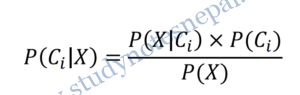Here, P(X) is constant for all classes. So, only P(X|Ci) × P(Ci) needs to be maximized.

It would be extremely computationally expensive to compute P(X|Ci). In order to reduce computation in evaluating P(X|Ci), the naïve assumption of class conditional independence is made. This presumes that the values of the attributes are conditionally independent of one another, given the class label of the tuple (i.e., that there are no dependence relationships among the attributes). Thus,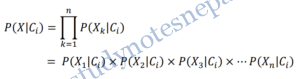For example,

Test data: X:(Age=Youth, Income=Medium, Student=Yes, Credit_Rating=Fair)
So,
To compute, ?(?|?? ) for i=1,2 we first compute following conditional probabilities:
P(Age = Youth | Buys_Computer = Yes) = 2/9 = 0.222
P(Age = Youth | Buys_Computer = No) = 3/5 = 0.600
P(Income = Medium | Buys_Computer = Yes) = 4/9 = 0.444
P(Income = Medium | Buys_Computer = No) = 2/5 = 0.400
P(Student = Yes | Buys_Computer = Yes) = 6/9 = 0.667
P(Student = Yes | Buys_Computer = No) = 1/5 = 0.200
P(Credit_Rating = Fair | Buys_Computer = Yes) = 6/9 = 0.667

P(Credit_Rating = Fair | Buys_Computer = No) = 2/5 = 0.400
Hence,
P(X|C1)=P(X| Buys_Computer=Yes)= P(Age = Youth | Buys_Computer = Yes) × P(Income = Medium | Buys_Computer = Yes) ×P(Student = Yes | Buys_Computer = Yes) ×P(Credit_Rating = Fair | Buys_Computer = Yes)
= 0.222 × 0.444 × 0.667 × 0.667
= 0.044

P(X|C2)=P(X| Buys_Computer=No) = P(Age = Youth | Buys_Computer = No) × P(Income = Medium | Buys_Computer = No) × P(Student = Yes | Buys_Computer = No) × P(Credit_Rating = Fair | Buys_Computer = No)
= 0.600 × 0.400 × 0.200 × 0.400
= 0.019

To find class Ci that maximizes P(X|Ci)P(Ci), we compute,

Therefore Naïve Bayesian Classifier classifies
X:(Age=Youth, Income=Medium, Student=Yes, Credit_Rating=Fair)

## 3.6 Artificial Neural Network Classifier

An Artificial Neural Network is an abstract computational model of the human brain. The human brain has an estimated 1011 tiny units called neurons. These neurons are interconnected with an estimated 1015 links. Similar to the brain, an ANN is composed of artificial neurons (or processing units) and interconnections. When we view such a network as a graph, neurons can be represented as nodes (or vertices) and interconnections as edges. Although the term ANN is most commonly used, other names include “neural network”, parallel distributed processing (PDP) system, connectionist model, and distributed adaptive system. ANNs are also referred to in the literature as neurocomputers.

An Artificial Neural Network (ANN) is a massive parallel distributed processor made up of simple processing units. It has the ability to learn experiential knowledge expressed through interunit connection strengths, and can make such knowledge available for use.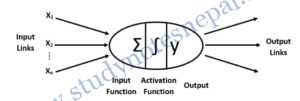ANN represents a very basic level to imitate the type of nonlinear learning that occurs in the nature. The inputs (x ) are collected from upstream neurons (or the data set) and combined through a combination function such as summation (Σ), which is then input into a (usually nonlinear) activation function to produce an output response (y), which is then channeled downstream to other neurons.

Backpropagation learns by iteratively processing a data set of training tuples, comparing the network’s prediction for each tuple with the actual known target value. For each training tuple, the weights are modified so as to minimize the mean squared error between the network’s prediction and the actual target value. These modifications are made in the “backwards” direction, that is, from the output layer, through each hidden layer down to the first hidden layer (hence the name backpropagation). Although it is not guaranteed, in general the weights will eventually converge, and the learning process stops.

Before training the network topology must be designed by:

• Specifying number of input nodes/units: Depends upon number of independent variable in data set.
• Specifying Number of hidden layers: Generally only one layer is considered in most of theproblem. Two layers can be designed for complex problem. Number of nodes in the hidden layer can be adjusted iteratively.
• Number of output nodes/units: Depends upon number of class labels of the data set.
• Learning rate: Can be adjusted iteratively.
• Learning algorithm: Any appropriate learning algorithm can be selected during training phase.
• Bias value: Can be adjusted iteratively.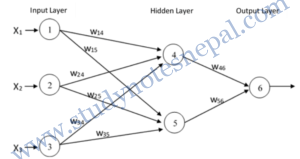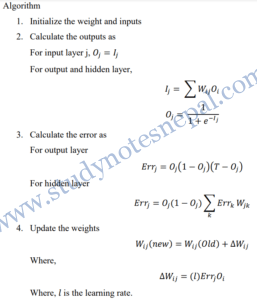• High tolerance of noisy data
• Classify patterns on which they have not been trained
• Can be used in various applications such as handwriting recognition, image classification, text narration etc.
• Parallelization can be implemented

• Require long training time
• Requires number of parameters whose best value is unknown
• Difficulty to interpret the meaning of weights and hidden network

## 3.7 Issues: Overfitting, Validation and Model Comparison

### Overfitting

Overfitting refers to a model that models the training data too well. Overfitting occurs when a statistical model describes random error or noise instead of the underlying relationship. This means that the noise or random fluctuations in the training data is picked up and learned as concepts by the model. The problem is that these concepts do not apply to new data and negatively impact the models ability to generalize.

Overfitting generally occurs when a model is excessively complex, such as having too many parameters relative to the number of observations. A model which has been overfit will generally have poor predictive performance. In order to avoid Overfitting, it is necessary to use additional techniques (e.g. cross validation, pruning (Pre or Post), model comparison etc.

Reason
– Noise in training data.
– Incomplete training data.
– Flaw in assumed theory

### Underfitting:

It refers to a model that can neither model the training data nor generalize to new data. An underfit machine learning model is not a suitable model and will be obvious as it will have poor performance on the training data. Underfitting is often not discussed as it is easy to detect given a good performance metric. The remedy is to move on and try alternate machine learning algorithms.

How to Limit Overfitting

Both Overfitting and underfitting can lead to poor model performance. But by far the most common problem in applied machine learning is overfitting.

Overfitting is such a problem because the evaluation of machine learning algorithms on training data is different from the evaluation we actually care the most about, namely how well the algorithm performs on unseen data.

There are two important techniques that you can use when evaluating machine learning algorithms to limit overfitting:

• Use a resampling technique to estimate model accuracy.
• Hold back a validation dataset.

### Validation

Validation is the process of evaluating the model using the training dataset. It is done by a resampling techinique called cross validation.

Cross validation (The holdout method)

Datasets can be categorized into three types: the training data, the validation data, and the test data. The training data is used by one or more learning methods to come up with classifiers. The validation data is used to optimize parameters of those classifiers, or to select a particular one. Then the test data is used to calculate the error rate of the final, optimized, method.

The real problem occurs when there is not a vast supply of data available. In many situations the training data must be classified manually—and so must the test data, of course, to obtain error estimates. This limits the amount of data that can be used for training, validation, and testing, and the problem becomes how to make the most of a limited dataset. From this dataset, a certain amount is held over for testing—this is called the holdout procedure—and the remainder is used for training (and, if necessary, part of that is set aside for validation).

k-fold cross-validation
In k-fold cross-validation, the initial data are randomly partitioned into k mutually exclusive subsets or “folds,” D1, D2 . . . , Dk, each of approximately equal size. Training and testing is performed k times. In iteration i, partition Di is reserved as the test set, and the remaining partitions are collectively used to train the model. That is, in the first iteration, subsets D2, . . . , Dk collectively serve as the training set in order to obtain a first model, which is tested on D1; the second iteration is trained on subsets D1, D3, . . . , Dk and tested on D2; and so on. Unlike the holdout and random
ubsampling methods above, here, each sample is used the same number of times for training and
nce for testing. For classification, the accuracy estimate is the overall number of correct
lassifications from the k iterations, divided by the total number of tuples in the initial data.

### Model Comparison

A confusion matrix, sometimes called a classification matrix, is used to assess the prediction accuracy of a model. It measures whether a model is confused or not, that is, whether the model is making mistakes in its predictions or not.

In the two-class case with classes yes and no, buys computer or not, plays golf or not and so on, a single prediction has the four different possible outcomes shown in Table 3. Given m classes, a confusion matrix is a table of at least size m by m. An entry, CMi, j in the first m rows and m columns indicates the number of tuples of class i that were labeled by the classifier as class j.

True positive (TP) refer to the positive tuples that were correctly labeled by the classifier.

True Negative (TN) are the negative tuples that were correctly labeled by the classifier.

A false positive (FP) occurs when the outcome is incorrectly predicted as yes (or positive) when it is actually no (negative). e.g., tuples of class buys_computer = no for which the classifier predicted buys_computer = yes

A false negative (FN) occurs when the outcome is incorrectly predicted as negative when it is actually positive. e.g., tuples of class buys_computer = yes for which the classifier predicted buys_computer = no

Accuracy is not always the best measure of the quality of the classification model. It is especially true for the real – world problems where the distribution of classes is unbalanced. For example, if the problem is classification of healthy persons from those with the disease. In many cases the medical database for training and testing will contain mostly healthy persons (99%), and only small percentage of people with disease (about 1%). In that case, no matter how good the accuracy of a model is estimated to be, there is no guarantee that it reflects the real world. Therefore, we need other measures for model quality. In practice, several measures are developed, and some of the best known are as follows: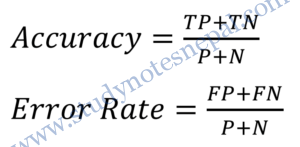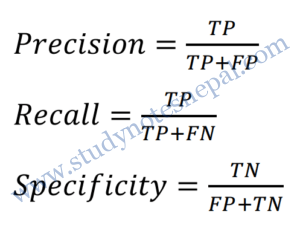—Refer notes for example—

### ROC Analysis

ROC curves are a useful visual tool for comparing two classification models. The name ROC stands for Receiver Operating Characteristic. A ROC curve shows the trade-off between the true positive rate or sensitivity (proportion of positive tuples that are correctly identified) and the falsepositive rate (proportion of negative tuples that are incorrectly identified as positive) for a given model. That is, given a two-class problem, it allows us to visualize the trade-off between the rate at which the model can accurately recognize ‘yes’ cases versus the rate at which it mistakenly identifies ‘no’ cases as ‘yes’ for different “portions” of the test set. Any increase in the true positive rate occurs at the cost of an increase in the false-positive rate.

The area under the ROC curve is a measure of the accuracy of the model. A typical example is a diagnostic process in medicine, where it is necessary to classify the patient as being with or without disease. For these types of problems, two different yet related error rates are of interest. The False Acceptance Rate (FAR) is the ratio of the number of test cases that are incorrectly “accepted” by a given model to the total number of cases. For example, in medical diagnostics, these are the cases in which the patient is wrongly predicted as having a disease. On the other hand, the False Reject Rate (FRR) is the ratio of the number of test cases that are incorrectly “rejected” by a given model to the total number of cases.

In order to plot an ROC curve for a given classification model, M, the model must be able to return a probability or ranking for the predicted class of each test tuple. That is, we need to rank the test tuples in decreasing order, where the one the classifier thinks is most likely to belong to the positive or ‘yes’ class appears at the top of the list. The vertical axis of an ROC curve represents the true positive rate. The horizontal axis represents the false-positive rate. An ROC curve for M is plotted as follows. Starting at the bottom left-hand corner (where the true positive rate and false-positive rate are both 0), we check the actual class label of the tuple at the top of the list. If we have a true positive (that is, a positive tuple that was correctly classified), then on the ROC curve, we move up and plot a point. If, instead, the tuple really belongs to the ‘no’ class, we have a false positive.

On the ROC curve, we move right and plot a point. This process is repeated for each of the test tuples, each time moving up on the curve for a true positive or toward the right for a false positive. To assess the accuracy of a model, we can measure the area under the curve. The closer the area is to 0.5, the less accurate the corresponding model is. A model with perfect accuracy will have an area of 1.0.

References
 D. T. LAROSE, DISCOVERING KNOWLEDGE IN DATA An Introduction to Data Mining, New Jersey: John Wiley & Sons, Inc., 2005.
 J. Han and K. Micheline, Data Mining: Concepts and Techniques, San Francisco: Elsevier Inc., 2006.
 P.-N. Tan, M. Steinbach and V. Kumar, INTRODUCTION TO DATA MINING, New York: PEARSON Addison Wesley, 2006.
 S. Chakrabarti, E. Cox, E. Frank, R. H. Guting, j. Han, X. Jiang and M. Kamber, Data Mining know It All, Burlington: Elsevier Inc, 2009.
 I. H. Witten and E. Frank, Data Mining Practical Machine Learning Tools and Techniques.